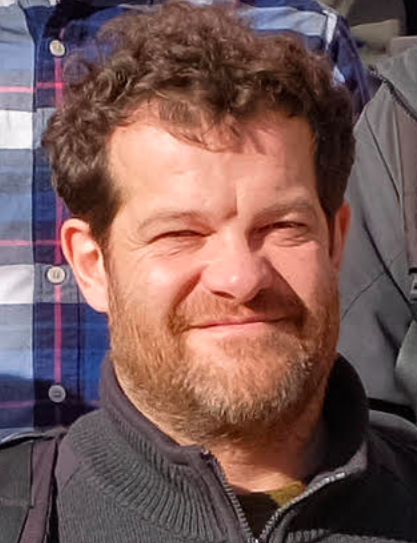# Invited Talks

Invited speakers

1. Marc NoyDepartment of Mathematics, Universitat Politècnica de Catalunya, Barcelona, Spain & Barcelona Graduate School of Mathematics, Barcelona, Spain

TitleLogic of sparse random graphs

Abstract: We survey recent results on limiting probabilities of graph properties expressible in first order logic and monadic second order logic for two models of sparse random graphs: the classical model G(n,p) with p = c/n, and random planar graphs and related classes of graphs under the uniform distribution.Theoretical Computer Science at the Institute of Mathematical Sciences, Chennai, India & Computer Science at University of Bergen, Norway

Title: Parameterized algorithms for geometric graphs via decomposition theorems

Abstract: Parameterized complexity is one of the most established algorithmic paradigms to deal with computationally hard problems. In the first two decades, the field largely focused on problems arising  from studies of graphs and networks. However, lately the focus has changed substantially and it has started to permeate into other fields such as computational geometry, and computational social choice theory. In this talk, we will survey some exciting developments in the emerging field of parameterized  computational geometry through  our contributions. We will focus on designing efficient parameterized algorithms on unit-disk graphs via new graph decomposition theorems.CNRS, projet COATI, commun I3S (CNRS, Univ. Nice-Sophia Antipolis), Sophia Antipolis, France & INRIA (UR Sophia Antipolis), Sophia Antipolis, France

TitleUnavoidability and universality of digraphs

Abstract: A digraph $F$ is {\it $n$-unadoidable} (resp. {\it $n$-universal}) if it is contained in every tournament of order $n$ (resp. $n$-chromatic digraph). Well-known theorems imply that there is an $n_F$ such that $F$ is $n_F$-unavoidable (resp. {\it $n_F$-universal}) if and only if $F$ is acyclic, (resp. an oriented forest). However, determining the smallest $n_F$ for which it occurs is a challenging question. In this talk, we survey the results on unavoidability and universality with an emphasis on oriented forests. In particular, we shall detail the following new results obtained jointly with F. Dross:  every arborescence of order $n$ with $k$ leaves is $(n+k-1)$-unavoidable, $(\frac{21}{8} n- \frac{47}{16})$-unavoidable, and $(n+ 144k^2 - 280k + 124)$-unavoidable.